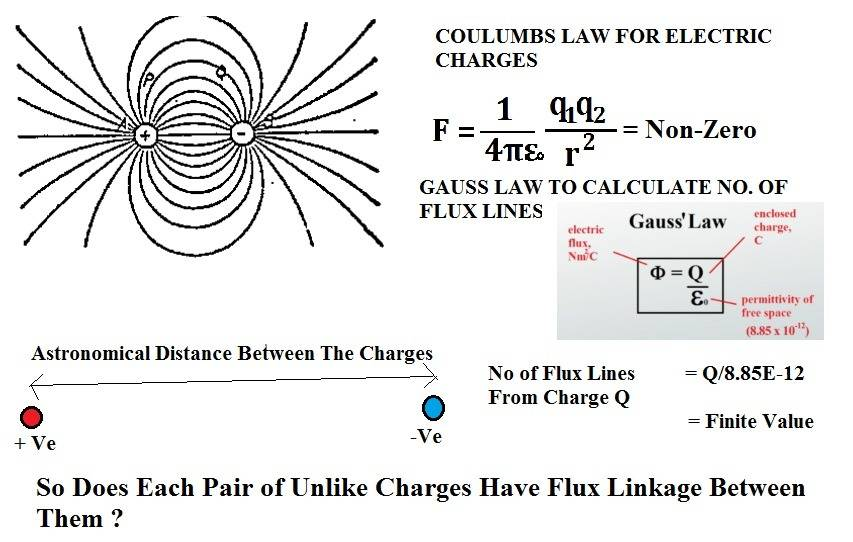# At least One Faraday Tube Between Every Two Unlike Charges in the Universe

Narayanan KRBy Classic Coulomb's Law there exists negligible yet non zero force of attraction between two unlike charges in-spite of the distance.
However for electrostatic attraction to work we need at-least one Faraday Tube(Lines of Forces) between the attracting charges, does that means,
1.A single positive charge is connected to all other negative charge in the universe via Line(s) of Forces and vice versa
2. A count of number of flux lines leaving a positive charge will give number of negative charges in the universe, but such will be an Infinite number, then why Gauss's formula gives a finite number of flux lines for a given charge ?
3. With number of flux lines of a charge being constant there will be many possible pairs of unlike charges in the universe that are completely isolated from each other and have exact zero coulumb's force between them.

••Delta2 and davenn

2022 Award
"Lines of flux" as drawn in your diagram are a visualisation tool. They are integral curves of the field, and infinitely many of them could be drawn in principle. ##\Phi## is often casually described as the number of field lines passing through a surface, and if you draw a finite number of lines and restrict yourself to surfaces perpendicular to the electric field then it's pretty close to proportional. But it's clearly not actually a literal count of field lines because it's not dimensionless.

Flux through an area is defined as ##\Phi=\int\vec E\cdot d\vec A##. Since ##\vec E## is everywhere defined, you can construct a small area that avoids any lines of flux you have drawn, but still have a non-zero ##\Phi##.

In short, you are over-interpreting a useful visualisation and aid to intuition as a fundamental law. You might be interested in reading my Insight article on the related topic of magnetic field lines.

Last edited:
•DaveE, Delta2, Narayanan KR and 2 others
Narayanan KR
"Lines of flux" as drawn in your diagram are a visualisation tool. They are integral curves of the field, and infinitely many of them could be drawn in principle. ##\Phi## is often casually described as the number of field lines passing through a surface, and if you draw a finite number of lines and restrict yourself to surfaces perpendicular to the electric field then it's pretty close to proportional. But it's clearly not actually a literal count of field lines because it's not dimensionless.

Flux through an area is defined as ##\Phi=\int\vec E\cdot d\vec A##. Since ##\vec E## is everywhere defined, you can construct a small area that avoids any lines of flux you have drawn, but still have a non-zero ##\Phi##.

In short, you are over-interpreting a useful visualisation and aid to intuition as a fundamental law. You might be interested in reading my Insight article on the related topic of magnetic field lines.

•Ibix
Homework Helper
Gold Member
Hard to explain where you go wrong in 1-3, in short it is what @Ibix said that you are over-interpreting the field lines tool as a fundamental law. Anyway I 'll try to state my view:

Gauss's law give us the number of field lines from a charge distribution, AS IF that charge distribution was the only one in the universe. That's why it will always give us the same number (as long as we don't change the enclosed charge distribution) regardless of what's happening in the rest of the universe.

So having in mind that, it doesn't mean that because the flux we get from Gauss's law is constant that the coulomb force between some two opposite charges in the universe is zero, and neither 2. will hold because Gauss's law simply doesn't count the field lines as you think it does. Gauss's law counts the field lines as if the enclosed charge was the only charge in the universe (ok i am repeating myself here, sorry).

•Narayanan KR
Keith_McClary
If (on the z axis) you have positive charges at +1 , -1 and negative charges at 2 , -2, then there are no lines of force crossing the z=0 plane.

•Narayanan KR
Narayanan KR
Yes, but the Electric Field E or the potential V at z= -2 (say) will be still a function of both z=1 and z=2